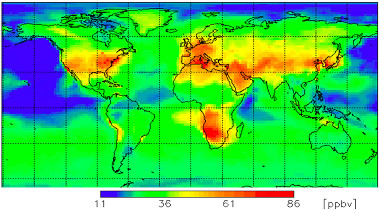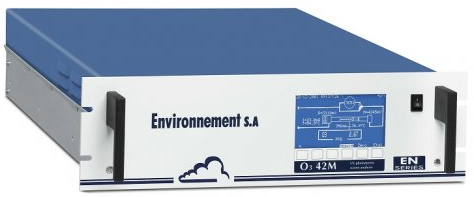﻿臭氧AQI：使用的濃度是毫克還是 ppb ？
 首頁 這裡 地圖 口罩！ 常見問題 搜索 聯繫 鏈接

# 臭氧AQI：使用的濃度是毫克還是 ppb ？

Posted on September 6th 2015
Share: aqicn.org/faq/2015-09-06/ozone-aqi-using-concentrations-in-milligrams-or-ppb/hk/

The ambient Ozone, refered as O3 and also called called ground-level or tropospheric Ozone impacts everyone on earth regardless of the country, as shown on the image on the right .

Afternoon ground level Ozone concentration in July 2011(Attribution: WMO GAW research on reactive gases )

Unlike particulate matter (PM2.5), the ground-level Ozone is not emitted directly. It is instead produced through a series of chemical reactions that occur in presence of nitrogen oxides, volatile organic compounds, sunlight and high temperatures, as shown on the following visual:Quantifying the impact of this ground level Ozone on Health is done via the Air Quality Index standard which each countries defines. What is interesting is that half of the world is using a standard based on milligrams measurement, while the rest is using ppb based measurement. But is this really a problem? This is what we will be looking at in this article.

--

The US EPA standard for Ozone is based on ppm, while the European one are based on milligrams.So, we naturally targetted our question to Environnement S.A, one of the leading European enviromental equipment manufacturer, who develops its own O342M Ozone analyser (see picture on the right). The O342M is both both certified by the US EPA and the EU (see specsheet), therefore able to provide measurement in both ppm and milligrams. Our question to Environnement S.A was 'How does your ozone analyser support both ppm and milligrams output? Is there any hardware difference for measurement? If not, is there any standard for the convertion?'.

## Ozone Measurement PrinciplesThe ozone measurement is based on the universally known UV absorption principle , which consists in measuring UV absorption of ozone molecules. Ozone concentration is determined by difference between UV absorption of the gas sample and the sample without ozone after filtration performed by a catalytic converter.

In this system, the Ozone concentration is measured as an amount of light energy per volume of air, from which the concentration in ppbv is deducted. The lower detectable limit for this system is 0.4 ppb (corresponding to an AQI of 0.3, based on the US EPA 8-hour Ozone standard). This system does not measure the mass as such, but there is a standard way of doing the convertion from ppmv to mg/m3.

## Converting Atmospheric Pollutant Concentrations: from ppmv to mg/m3

First, ppm (parts per million  ) and ppb (parts per billion) are defined as 1 ppm = 1/106 = 10-6 and 1 ppb = 1/109 = 10-9. So 1 ppm = 1000 ppb or 1 ppb = 10-3 ppm.

The conversion factor depends on the temperature at which you want the conversion (usually 25 degrees Centigrade in the US), as well as the ambient pressure. At an ambient pressure of 1 atmosphere, the general equation is:

$$c = { ppmv \times 12.187 \times MW \over 273.15 + t }$$
where:
• c = concentration in mg/m3(i.e., milligrams of gaseous pollutant per cubic meter of ambient air)
• MW = molecular weight of the gaseous pollutant
• ppmv = parts per million by volume (i.e., volume of gaseous pollutant per million volumes of ambient air)
• t = ambient temperature in degrees centigrade.
• 12.187 = inverse of the Universal Gas Law constant
As an example, for gaseous pollutant O3 (ozone), to convert 20 ppmv of Ozone to mg/m3 at 25 °C and 1 atmophere, the following formula is used:

$$c(20 ppmv) = { 20 \times 12.187 \times 48 \over 273.15 + 25 } = {20 \times 1.97} = 39.4 mg/m^3$$

where: 48.00 = MW(O3) = molecular weight of Ozone O3

## European and US Convertion Standards

The assumption on the ambient temperature and atmospheric pressure is actually standardized and summurized in the following table for US, Europe or normal conditions. For our O342M Ozone analyzers, this coefficient is user programmable by the operator of the instrument.

 Gas Standard Conditions for Temperature and Pressure ( STP) "STP US" Conditions at 25°C (US EPA standard)  1013 mbar and 298K "STP European Union" Conditions at 20°C (EU standard)  1013 mbar and 293K "Normal" Conditions at 0°C 1013 mbar and 273K O3 - Ozone 1 ppb = 1,97 µg/m3 1 ppb = 2,00 µg/m3 1 ppb = 2,15 µg/m3 NO2 - Nitrogen Dioxyde 1 ppb = 1,88 µg/m3 1 ppb = 1,91 µg/m3 1 ppb = 2,05 µg/m3

Note: For those interested in knowing why 20°C is used as the standard reference temperature, you can check Ted Doiron's article on "20 °C—A short history of the standard reference temperature for industrial dimensional measurements".
Many thanks to Serge from Environnement S.A for the quick and precise answer on the Ozone monitors. Note that the same concept also applies to other gases such as Nitrogen Dioxide (for instance using the AS32M analyser).

--

To push the investigation a bit further, the next question is what would be the impact on the calculated Air Quality Index if the actual temperature and pressure would be used instead of the reference one?

## Impact from ambient temperature

The last question is to look at the impact of a temperature variation on the Air Quality Index. For instance, let assume that an instrument is measuring an average of 120 mg/m3 of Ozone over 1 hour, which corresponds to an AQI of 50 (Medium) according to the European Common Air Quality Index (CAQI).

At 20°C and 1 atm, 120 mg/m^3 converts to 120/2.00, i.e. 60.0 ppmv. So let us assume that this is the actual measurement from the Ozone sensor. The question is then, what if the ambient temperature was peaking to 42°C, as it sometimes happen during summer heat waves, then what would be the correct mass? The convertion formula is:

$$c = { ppmv \times 12.187 \times MW \over 273.15 + t } = 111.37$$
This results in a difference of 8.6 mg/m^3 of measured Ozone. When applying the CAQI standard, the corresponding AQI is 46.4 (instead of 50 using the standard 20°C condition). This is actually an acceptable difference.

The generalized adjustement formula depending on the ambient temperature is summurized with the graph on the right. The x-axis is the ambient temperature, and the y-axis the calculated AQI is the actual temperature would be used instead of the reference one (20°C).

## Impact from atmospheric pressure

As concerns the atmospheric pressure, the varation is defined by the Ideal Gas Law (PV = nRT). The value 12.187 is actually the inverse of the Universal Gas Law constant R. So, to understand the impact of atmospheric pressure, the following formula can be used:

$$R = {{ P \times V } \over {n \times T}} = {{ P \times 22.4 } \over { 1 \times 273 }} = { P \over 12.1875 }$$
In other words, one just need to divide the convertion factor by the current atmosphere. Assuming that the pressure p is expressed in millibars (1 atm = 1013.25 mb), the generalized convertion formula becomes:

$$c = { ppmv \times MW \times 1013.25 \times 12.1875 \over { p \times (273.15 + t)} }$$

where:
• c = concentration in mg/m3(i.e., milligrams of gaseous pollutant per cubic meter of ambient air)
• MW = molecular weight of the gaseous pollutant
• ppmv = parts per million by volume (i.e., volume of gaseous pollutant per million volumes of ambient air)
• t = ambient temperature in degrees centigrade.
• p = ambient atmospheric pressure in millibars.

## Conclusions

The above explanations confirm our initial hypothesis that even though Ozone readings can be provided with different units (ppm and mg/m^3), this is actually not a problem since there is a standard way to convert readings from mg/m^3 to ppm and vice-versa. Moreover, the impact of using the reference STP (Standard Temperature & Pressure) instead of the actual ambient temperature and pressure is minimal, i.e. just units of Index in difference for the Ozone.

Credits: Ozone visual recreated using Icon pack by Icons8 and taken from American Chemical Society.

 This Ozone is not to be confused with the good ozone also called stratospheric ozone
 Parts per million also can be expressed as milligrams per liter (mg/L). This measurement is the mass of a chemical or contaminate per unit volume of water. Seeing ppm or mg/L on a lab report means the same thing. from University of Minnesota
 https://en.wikipedia.org/wiki/Gas_constant

Click here to see all the FAQ entries
• AQI量表：顏色和數字是什麼意思？
• 使用統計距離進行實時傳感器網絡驗證
• 克里格插值

• Read or publish comments (they rely on Disqus)

# 關於空氣質量與空氣污染指數

本網站採用的污染指數和顏色與EPA是完全相同的。 EPA的指數可以從 AirNow上查到

 空气质量指数 空气质量指数级别（状况）及表示颜色 对健康影响情况 建议采取的措施 0 - 50 一级（优） 空气质量令人满意，基本无空气污染 各类人群可正常活动 51 -100 二级（良） 空气质量可接受，但某些污染物可能对极少数异常敏感人群健康有较弱影响 极少数异常敏感人群应减少户外活动 101-150 三级（轻度污染） 易感人群症状有轻度加剧，健康人群出现刺激症状 儿童、老年人及心脏病、呼吸系统疾病患者应减少长时间、高强度的户外锻炼 151-200 四级（中度污染） 进一步加剧易感人群症状，可能对健康人群心脏、呼吸系统有影响 儿童、老年人及心脏病、呼吸系统疾病患者避免长时间、高强度的户外锻炼，一般人群适量减少户外运动 201-300 五级（重度污染） 心脏病和肺病患者症状显著加剧，运动耐受力降低，健康人群普遍出现症状 儿童、老年人及心脏病、肺病患者应停留在室内，停止户外运动，一般人群减少户外运动 300+ 六级（严重污染） 健康人群运动耐受力降低，有明显强烈症状，提前出现某些疾病 儿童、老年人和病人应停留在室内，避免体力消耗，一般人群避免户外活动
(参考详见http://zh.wikipedia.org/wiki/空气质量指数)

如果你想了解更多有關空氣質量與污染，詳見維基百科或者 AirNow

有關健康建議詳​​見北京的Richard Saint Cyr MD醫生的博客：www.myhealthbeijing.com

注意事項: 所有空氣品質數據在發佈時均未經驗證，且為了確保資料準確性，這些數據可能隨時被修改，恕不另行通知。 世界空氣品質指數專案在編制這些訊息內容時已經謹慎的運用了所有適當的技巧，在任何情況下世界空氣品質指數在任何情況下，世界空氣品質指數專案團隊或其代理人均不對因提供此數據而直接或間接引起的任何損失、傷害或損害來承擔契約、侵權或其他責任。

## 設定

選擇語言：

 Temperature unit:Celcius